## Equinumerous

Letandbe two classes of Positive integers. Letbe the number of integers inwhich are less than or equal to, and let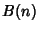be the number of integers inwhich are less than or equal to. Then if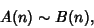andare said to be equinumerous.

The four classes of Primes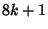,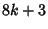,,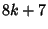are equinumerous. Similarly, sinceandare both of the form, andandare both of the form,andare also equinumerous.